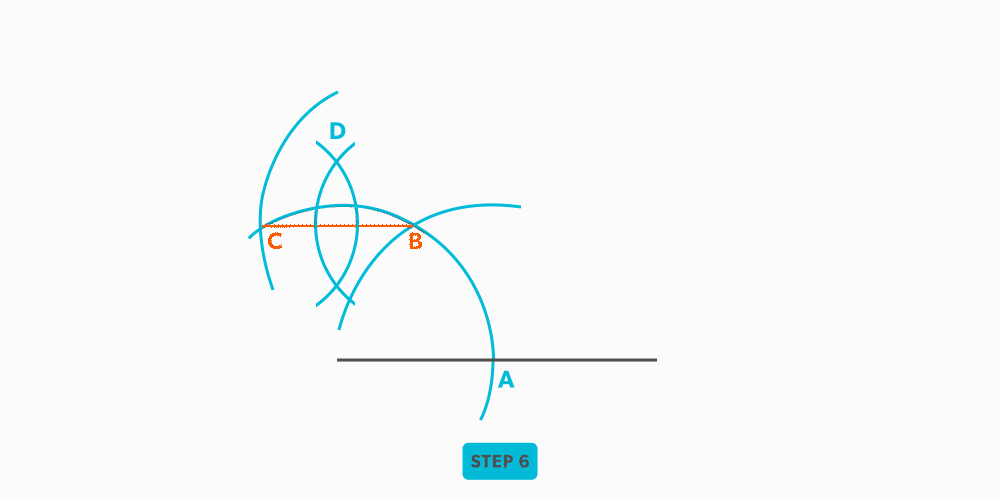Geometric Construction

# Create a Perpendicular Line at the Middle of a Line

1. Draw a line.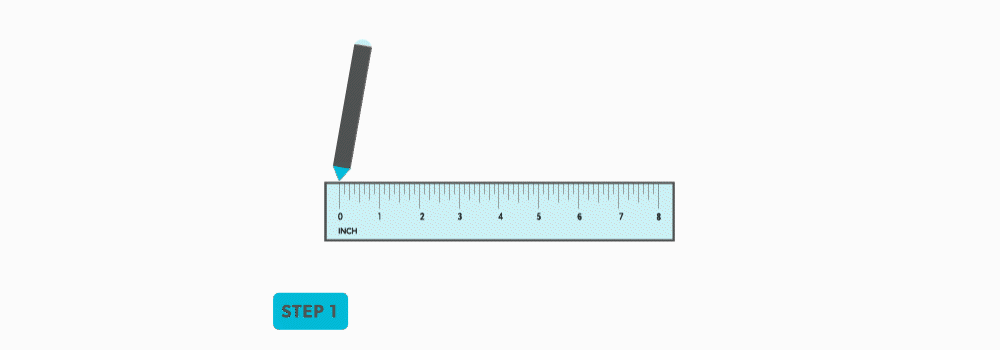2. the line.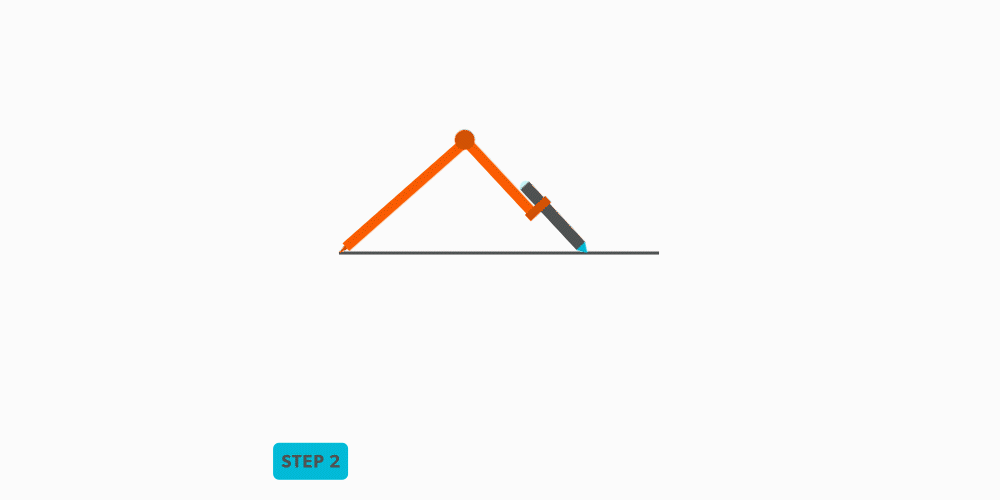3. In bisecting the line, we have created a line (x-y axis).# Create a Perpendicular Line at the End of a Line

1. Draw a line.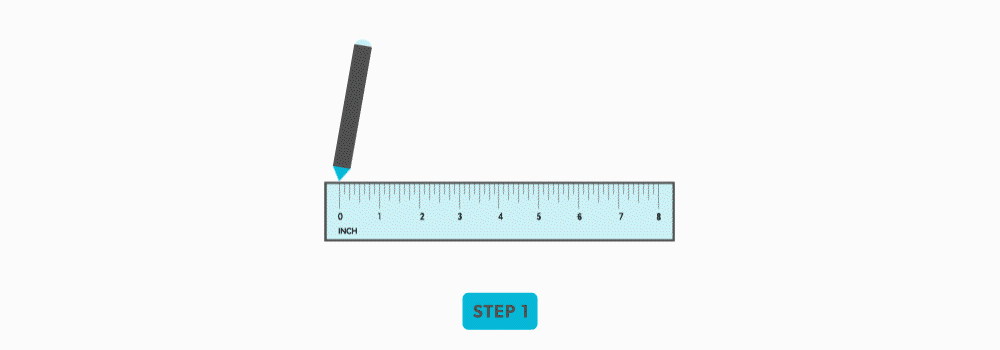2. Set your compass to a of reasonable distance.3. Swing that arc from the end of the line.4. Using the same distance (radius) on your compass, swing that distance along the arc twice. Each time you swing the radius along its own arc/, it create a 60° angle and .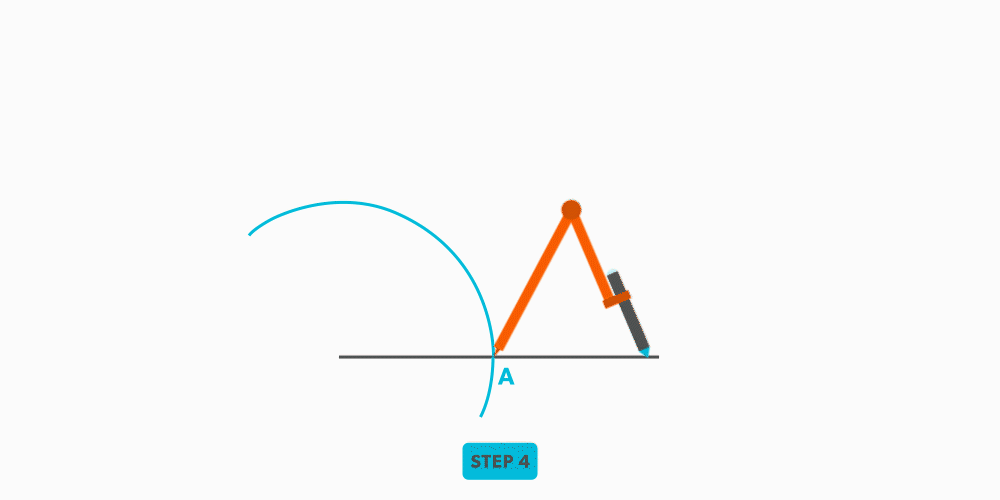5. Bisect the arc between the last two points. We are bisecting the second 60° sector to create two 30° sectors. If we then look at the arc, we have a 60° sector and a 30° sector, which combine to make a 90° .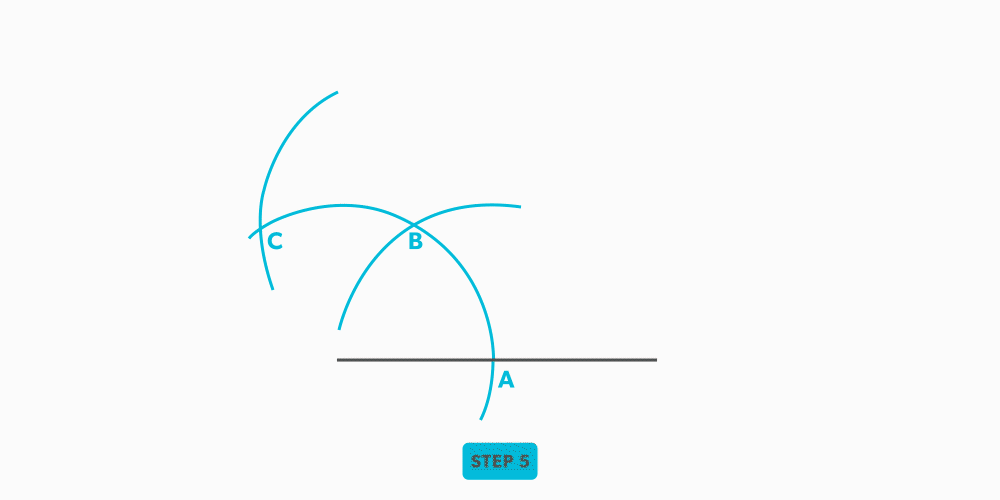6. From the end of the line, draw a line through the last point.